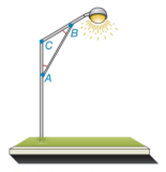Chapter 2.4, Problem 40EElementary Geometry For College St...

7th Edition
Alexander + 2 others
ISBN: 9781337614085

Solutions

Chapter
SectionElementary Geometry For College St...

7th Edition
Alexander + 2 others
ISBN: 9781337614085
Textbook Problem

For the lamppost of Exercise 39, Suppose that m ∠ A = m ∠ B and that m ∠ C = 3 ( m ∠ A ) . Find m ∠ A , m ∠ B and m ∠ C .Exercises 39 ,   40

To determine

To find:

The values of mA, mB and mC.

Explanation

Given:

mA=mB �and mC=3(mA)

Figure (1)

Approach:

The sum of interior angles of a triangle is equal to 180°.

Calculation:

mA+mB+mC=180°

Substitute 3(mA) for mC, and mA for mB�in equation (1)

Still sussing out bartleby?

Check out a sample textbook solution.

See a sample solution

The Solution to Your Study Problems

Bartleby provides explanations to thousands of textbook problems written by our experts, many with advanced degrees!

Get Started

let f(x) = x 1, g(x) = x+1, and h(x) = 2x3 1. Find the rule for each function. 11. fg

Applied Calculus for the Managerial, Life, and Social Sciences: A Brief Approach

State the domain and range of each of the function in Problems 25 – 28. 25.

Mathematical Applications for the Management, Life, and Social Sciences

Which is the best graph of r = 1 − sin θ for 0 ≤ θ ≤ π?

Study Guide for Stewart's Multivariable Calculus, 8th

The graph of x = cos t, y = sin2 t is:

Study Guide for Stewart's Single Variable Calculus: Early Transcendentals, 8th